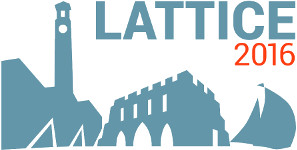#The 34th International Symposium on Lattice Field Theory (Lattice 2016)

Jul 24 – 30, 2016
Highfield Campus, University of Southampton
Europe/London timezone

## Monte Carlo simulation of $\phi^4_2$ and $O(N)\,\phi^4_3$ theories

Jul 26, 2016, 4:30 PM
20m
Building 67 Room 1007 (Highfield Campus, University of Southampton)

### Building 67 Room 1007

#### Highfield Campus, University of Southampton

Talk Algorithms and Machines

### Speaker

Ms Barbara De Palma (Istituto Nazionale di Fisica Nucleare (INFN) and Università di Pavia)

### Description

We report lattice simulations of $\phi^4_2$ and $O(N)\,\phi^4$ models, performed by means a Monte Carlo method based on the all-order strong coupling expansion (worm algorithm). The investigation of the non–perturbative features of the $\phi^4$ continuum limit in two dimensions lead us to the result $g/\mu^2 = 11.15 \pm 0.06_{stat} \pm 0.03_{syst}$ for the critical coupling. Furthermore we present a study of the scaling behaviour of worm and loop size in two-dimensional $O(N)$ model (non-linear $\sigma$-model) and three-dimensional $\phi^4\,O(N)$ model.

### Primary authors

Ms Barbara De Palma (Istituto Nazionale di Fisica Nucleare (INFN) and Università di Pavia) Dr Marco Guagnelli (INFN Pavia)

 Slides